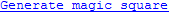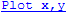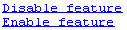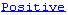## Create Hyperlinks that Run Functions

The special keyword `matlab:` lets you embed commands in other functions. Most commonly, the functions that contain it display hyperlinks, which execute the commands when you click the hyperlink text. Functions that support `matlab:` syntax include `disp`, `error`, `fprintf`, `help`, and `warning`.

Use `matlab:` syntax to create a hyperlink in the Command Window that runs one or more functions. For example, you can use `disp` to display the word Hypotenuse as an executable hyperlink as follows:

`disp('<a href="matlab:a=3; b=4;c=hypot(a,b)">Hypotenuse</a>')`
Clicking the hyperlink executes the three commands following `matlab:`, resulting in
```c = 5```
Executing the link creates or redefines the variables `a`, `b`, and `c` in the base workspace.

The argument to `disp` is an `<a href>` HTML hyperlink. Include the full hypertext text, from `'<a href=` to `</a>'` within a single line, that is, do not continue long text on a new line. No spaces are allowed after the opening `<` and before the closing `>`. A single space is required between `a` and `href`.

You cannot directly execute `matlab:` syntax. That is, if you type

`matlab:a=3; b=4;c=hypot(a,b)`
you receive an error, because MATLAB® interprets the colon as an array operator in an illegal context:
```??? matlab:a=3; b=4;c=hypot(a,b) | Error: The expression to the left of the equals sign is not a valid target for an assignment.```

You do not need to use `matlab:` to display a live hyperlink to the Web. For example, if you want to link to an external Web page, you can use `disp`, as follows:

`disp('<a href="http://en.wikipedia.org/wiki/Hypotenuse">Hypotenuse</a>')`
The result in the Command Window looks the same as the previous example, but instead opens a page at en.wikipedia.org:
`Hypotenuse`

Using `matlab:`, you can:

### Run a Single Function

Use `matlab:` to run a specified statement when you click a hyperlink in the Command Window. For example, run this command:

```disp('<a href="matlab:magic(4)">Generate magic square</a>') ```

It displays this link in the Command Window:When you click the link, MATLAB runs `magic(4)`.

### Run Multiple Functions

You can run multiple functions with a single link. For example, run this command:

`disp('<a href="matlab: x=0:1:8;y=sin(x);plot(x,y)">Plot x,y</a>')`

It displays this link in the Command Window:When you click the link, MATLAB runs this code:

```x = 0:1:8; y = sin(x); plot(x,y) ```

Redefine `x` in the base workspace:

`x = -2*pi:pi/16:2*pi;`

Click the hyperlink, `Plot x,y` again and it changes the current value of `x` back to `0:1:8`. The code that `matlab:` runs when you click the `Plot x,y` defines `x` in the base workspace.

### Provide Command Options

Use multiple `matlab:` statements in a file to present options, such as

```disp('<a href = "matlab:state = 0">Disable feature</a>') disp('<a href = "matlab:state = 1">Enable feature</a>') ```

The Command Window displays the links that follow. Depending on which link you click, MATLAB sets `state` to `0` or `1`.### Include Special Characters

MATLAB correctly interprets most text that includes special characters, such as a greater than symbol (`>`). For example, the following statement includes a greater than symbol (`>`).

`disp('<a href="matlab:str = ''Value > 0''">Positive</a>')`Some symbols might not be interpreted correctly and you might need to use the ASCII value for the symbol. For example, an alternative way to run the previous statement is to use ASCII 62 instead of the greater than symbol:

`disp('<a href="matlab:str=[''Value '' char(62) '' 0'']">Positive</a>')`